GROUP THEORY SYMBOL

# GTReciprocalBasis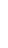GTReciprocalBasis[lattice vectors]
calculates the reciprocal lattice vectors from a given set of lattice vectors.

## DetailsDetails

• If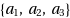are the lattice vectors and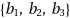are the corresponding reciprocal lattice vectors, the vectors fullfill the relation
•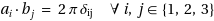• The module works for two and three dimensional lattices.
• Three dimensional reciprocal lattice vectors can be calculated by
•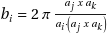(cyclic permutations of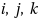)
• See: W. Hergert, M. Geilhufe, Group Theory in Solid State Physics and Photonics. Problem Solving with Mathematica, chapter 6.2

## ExamplesExamplesopen allclose all

### Basic Examples  (2)Basic Examples  (2)

First load the package:

 In:=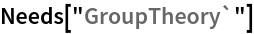The basis of a simple cubic lattice is given:

 In:=The reciprocal basis follows as :

 In:=Out=the condition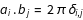has to be fulfilled.

 In:=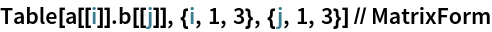Out//MatrixForm=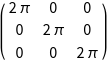For an fcc lattice we get:

 In:=Out=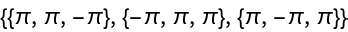In:=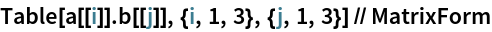Out//MatrixForm=In:=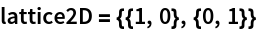Out=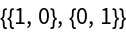In:=Out=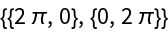## See AlsoSee Also

GTAbinitConvertWaveFunctionGTAnalyzeBandStructureGTGetStructureGTGroupOfKGTInstallStructureGTLatticeVectorsGTPwHamiltonian